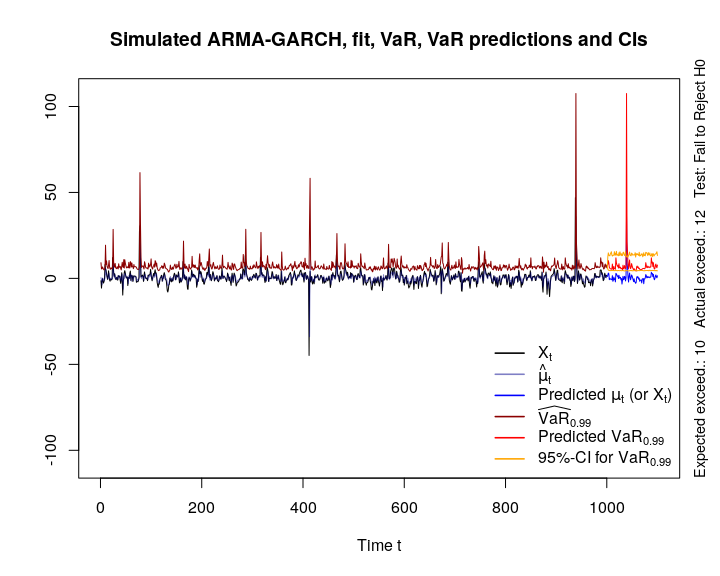# Fitting and Predicting VaR based on an ARMA-GARCH Process

#### 2020-01-13

This vignette does not use qrmtools, but shows how Value-at-Risk (VaR) can be fitted and predicted based on an underlying ARMA-GARCH process (which of course also concerns QRM in the wider sense).

library(rugarch)
library(qrmtools)

## 1 Simulate (-log-return) data $$(X_t)$$ from an ARMA-GARCH process

We consider an ARMA(1,1)-GARCH(1,1) process with $$t$$ distributed innovations.

## Model specification (for simulation)
nu <- 3 # d.o.f. of the standardized distribution of Z_t
fixed.p <- list(mu = 0, # our mu (intercept)
ar1 = 0.5, # our phi_1 (AR(1) parameter of mu_t)
ma1 = 0.3, # our theta_1 (MA(1) parameter of mu_t)
omega = 4, # our alpha_0 (intercept)
alpha1 = 0.4, # our alpha_1 (GARCH(1) parameter of sigma_t^2)
beta1 = 0.2, # our beta_1 (GARCH(1) parameter of sigma_t^2)
shape = nu) # d.o.f. nu for standardized t_nu innovations
armaOrder <- c(1,1) # ARMA order
garchOrder <- c(1,1) # GARCH order
varModel <- list(model = "sGARCH", garchOrder = garchOrder)
spec <- ugarchspec(varModel, mean.model = list(armaOrder = armaOrder),
fixed.pars = fixed.p, distribution.model = "std") # t standardized residuals

Simulate one path (for illustration purposes).

## Simulate (X_t)
n <- 1000 # sample size (= length of simulated paths)
x <- ugarchpath(spec, n.sim = n, m.sim = 1, rseed = 271) # n.sim length of simulated path; m.sim = number of paths
## Note the difference:
## - ugarchpath(): simulate from a specified model
## - ugarchsim():  simulate from a fitted object

## Extract the resulting series
X <- fitted(x) # simulated process X_t = mu_t + epsilon_t for epsilon_t = sigma_t * Z_t
sig <- sigma(x) # volatilities sigma_t (conditional standard deviations)
eps <- x@path$residSim # unstandardized residuals epsilon_t = sigma_t * Z_t ## Note: There are no extraction methods for the unstandardized residuals epsilon_t ## for uGARCHpath objects (only for uGARCHfit objects; see below). ## Sanity checks (=> fitted() and sigma() grab out the right quantities) stopifnot(all.equal(X, x@path$seriesSim, check.attributes = FALSE),
all.equal(sig, x@path$sigmaSim, check.attributes = FALSE)) As a sanity check, let’s plot the simulated path, conditional standard deviations and residuals. ## Plots plot(X, type = "l", xlab = "t", ylab = expression(X[t]))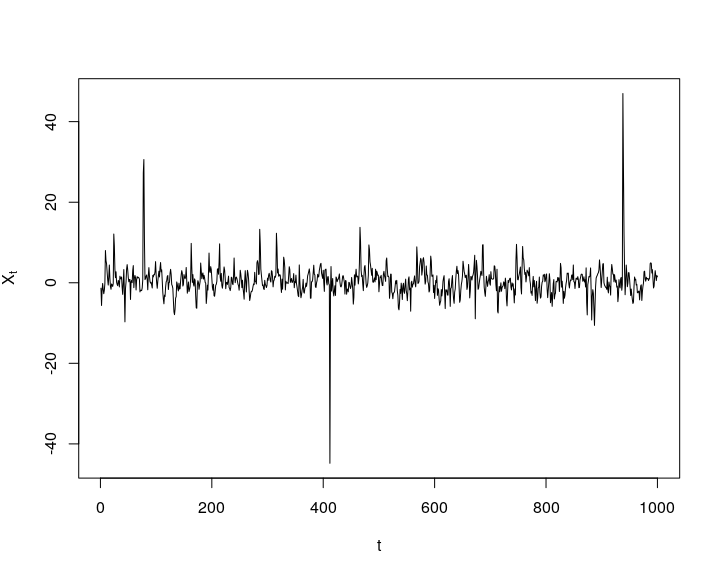plot(sig, type = "h", xlab = "t", ylab = expression(sigma[t]))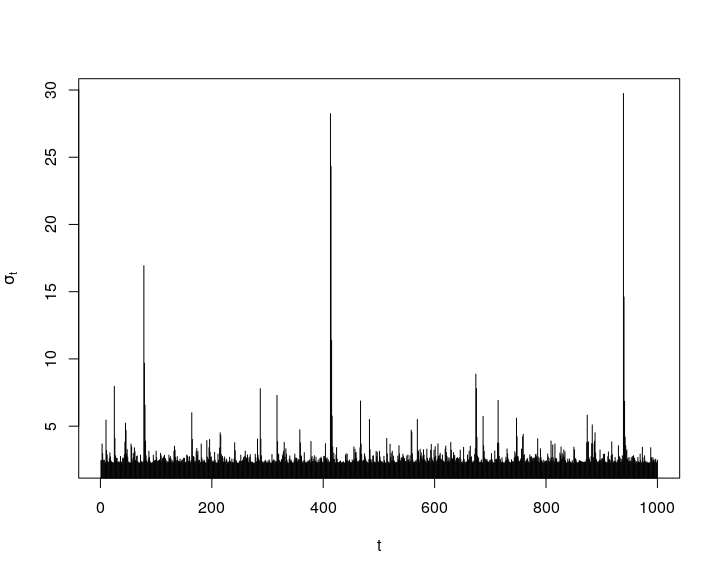plot(eps, type = "l", xlab = "t", ylab = expression(epsilon[t]))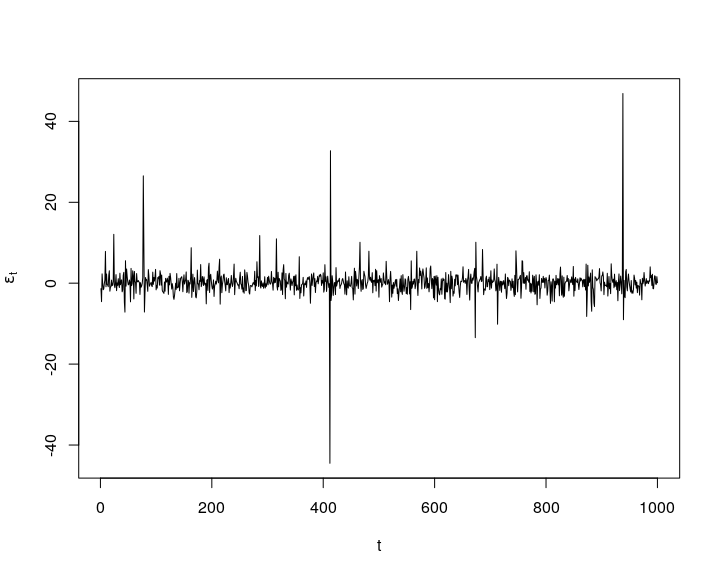## 2 Fit an ARMA-GARCH model to the (simulated) data Fit an ARMA-GARCH process to X (with the correct, known orders here; one would normally fit processes of different orders and then decide). ## Fit an ARMA(1,1)-GARCH(1,1) model spec <- ugarchspec(varModel, mean.model = list(armaOrder = armaOrder), distribution.model = "std") # without fixed parameters here fit <- ugarchfit(spec, data = X) # fit ## Extract the resulting series mu. <- fitted(fit) # fitted hat{mu}_t (= hat{X}_t) sig. <- sigma(fit) # fitted hat{sigma}_t ## Sanity checks (=> fitted() and sigma() grab out the right quantities) stopifnot(all.equal(as.numeric(mu.), fit@fit$fitted.values),
all.equal(as.numeric(sig.), fit@fit$sigma)) Again let’s consider some sanity checks. ## Plot data X_t and fitted hat{mu}_t plot(X, type = "l", xlab = "t", ylab = expression("Data"~X[t]~"and fitted values"~hat(mu)[t])) lines(as.numeric(mu.), col = adjustcolor("blue", alpha.f = 0.5)) legend("bottomright", bty = "n", lty = c(1,1), col = c("black", adjustcolor("blue", alpha.f = 0.5)), legend = c(expression(X[t]), expression(hat(mu)[t])))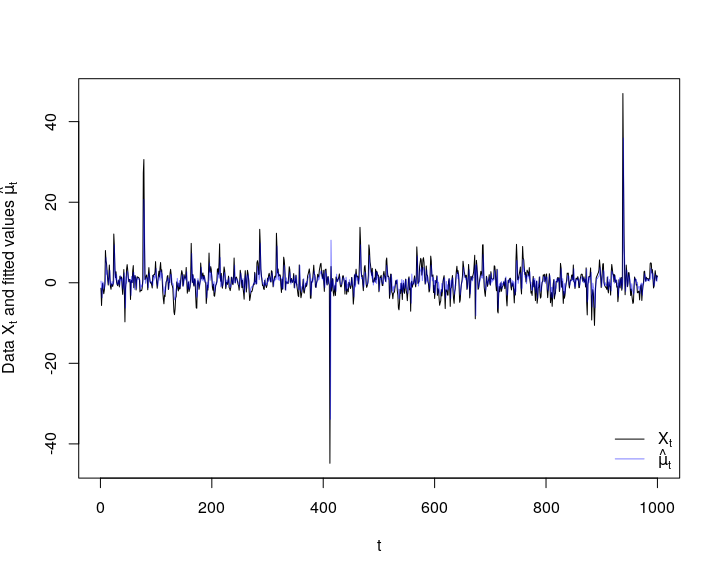## Plot the unstandardized residuals epsilon_t resi <- as.numeric(residuals(fit)) stopifnot(all.equal(fit@fit$residuals, resi))
plot(resi, type = "l", xlab = "t", ylab = expression(epsilon[t])) # check residuals epsilon_t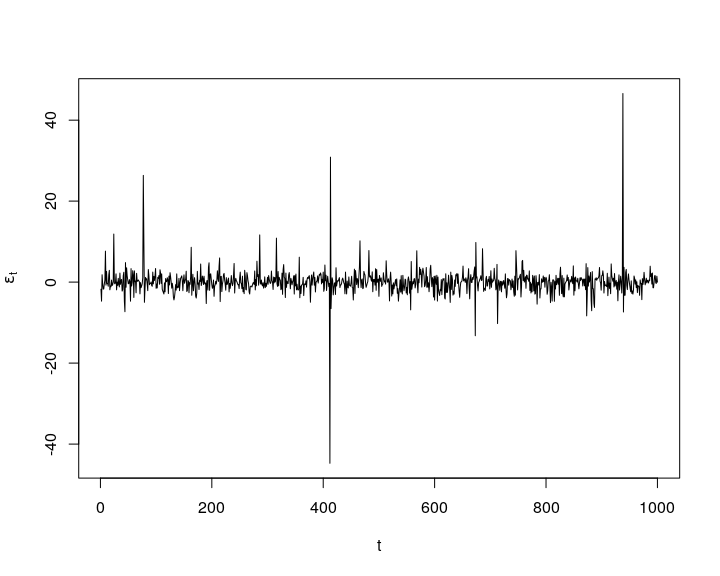## Q-Q plot of the standardized residuals Z_t against their specified t
## (t_nu with variance 1)
Z <- as.numeric(residuals(fit, standardize = TRUE))
stopifnot(all.equal(Z, fit@fit$z, check.attributes = FALSE), all.equal(Z, as.numeric(resi/sig.))) qq_plot(Z, FUN = function(p) sqrt((nu-2)/nu) * qt(p, df = nu), main = substitute("Q-Q plot of ("*Z[t]*") against a standardized"~italic(t)[nu.], list(nu. = round(nu, 2))))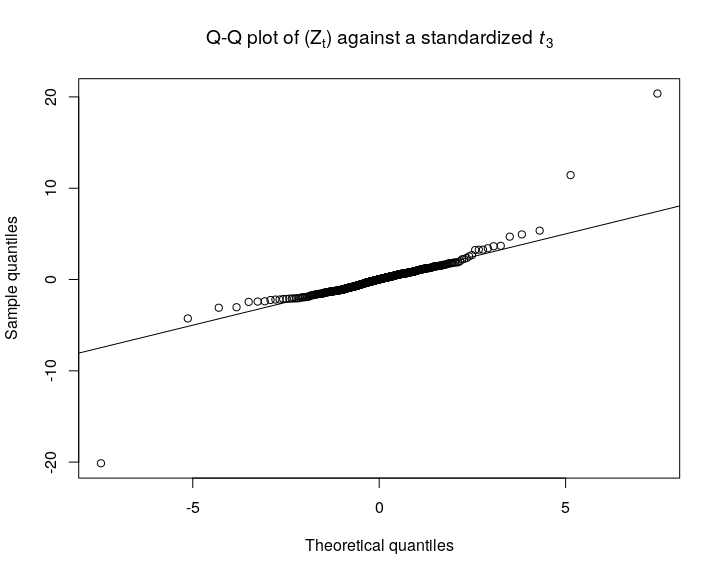## 3 Calculate the VaR time series Compute VaR estimates. Note that we could have also used the GPD-based estimators here. ## VaR confidence level we consider here alpha <- 0.99 ## Extract fitted VaR_alpha VaR. <- as.numeric(quantile(fit, probs = alpha)) ## Build manually and compare the two nu. <- fit@fit$coef[["shape"]] # extract (fitted) d.o.f. nu
VaR.. <- as.numeric(mu. + sig. * sqrt((nu.-2)/nu.) * qt(alpha, df = nu.)) # VaR_alpha computed manually
stopifnot(all.equal(VaR.., VaR.))
## => quantile(<rugarch object>, probs = alpha) provides VaR_alpha = hat{mu}_t + hat{sigma}_t * q_Z(alpha)

## 4 Backtest VaR estimates

Let’s backtest the VaR estimates.

## Note: VaRTest() is written for the lower tail (not sign-adjusted losses)
##       (hence the complicated call here, requiring to refit the process to -X)
btest <- VaRTest(1-alpha, actual = -X,
VaR = quantile(ugarchfit(spec, data = -X), probs = 1-alpha))
btest$expected.exceed # number of expected exceedances = (1-alpha) * n ##  10 btest$actual.exceed # actual exceedances
##  12
## Unconditional test
btest$uc.H0 # corresponding null hypothesis ##  "Correct Exceedances" btest$uc.Decision # test decision
##  "Fail to Reject H0"
## Conditional test
btest$cc.H0 # corresponding null hypothesis ##  "Correct Exceedances & Independent" btest$cc.Decision # test decision
##  "Fail to Reject H0"

## 5 Predict VaR based on fitted model

Now predict VaR.

## Predict from the fitted process
fspec <- getspec(fit) # specification of the fitted process
setfixed(fspec) <- as.list(coef(fit)) # set the parameters to the fitted ones
m <- ceiling(n / 10) # number of steps to forecast (roll/iterate m-1 times forward with frequency 1)
pred <- ugarchforecast(fspec, data = X, n.ahead = 1, n.roll = m-1, out.sample = m) # predict from the fitted process

## Extract the resulting series
mu.predict <- fitted(pred) # extract predicted X_t (= conditional mean mu_t; note: E[Z] = 0)
sig.predict <- sigma(pred) # extract predicted sigma_t
VaR.predict <- as.numeric(quantile(pred, probs = alpha)) # corresponding predicted VaR_alpha

## Checks
## Sanity checks
stopifnot(all.equal(mu.predict, pred@forecast$seriesFor, check.attributes = FALSE), all.equal(sig.predict, pred@forecast$sigmaFor, check.attributes = FALSE)) # sanity check
## Build predicted VaR_alpha manually and compare the two
VaR.predict. <- as.numeric(mu.predict + sig.predict * sqrt((nu.-2)/nu.) *
qt(alpha, df = nu.)) # VaR_alpha computed manually
stopifnot(all.equal(VaR.predict., VaR.predict))

## 6 Simulate future trajectories of $$(X_t)$$ and compute corresponding VaRs

Simulate paths, estimate VaR for each simulated path (note that quantile() can’t be used here so we have to construct VaR manually) and compute bootstrapped confidence intervals for $$\mathrm{VaR}_\alpha$$.

## Simulate B paths
B <- 1000
set.seed(271)
X.sim.obj <- ugarchpath(fspec, n.sim = m, m.sim = B) # simulate future paths

## Compute simulated VaR_alpha and corresponding (simulated) confidence intervals
## Note: Each series is now an (m, B) matrix (each column is one path of length m)
X.sim <- fitted(X.sim.obj) # extract simulated X_t
sig.sim <- sigma(X.sim.obj) # extract sigma_t
eps.sim <- X.sim.obj@path$residSim # extract epsilon_t VaR.sim <- (X.sim - eps.sim) + sig.sim * sqrt((nu.-2)/nu.) * qt(alpha, df = nu.) # (m, B) matrix VaR.CI <- apply(VaR.sim, 1, function(x) quantile(x, probs = c(0.025, 0.975))) ## 7 Plot Finally, let’s display all results. ## Setup yran <- range(X, # simulated path mu., VaR., # fitted conditional mean and VaR_alpha mu.predict, VaR.predict, VaR.CI) # predicted mean, VaR and CIs myran <- max(abs(yran)) yran <- c(-myran, myran) # y-range for the plot xran <- c(1, length(X) + m) # x-range for the plot ## Simulated (original) data (X_t), fitted conditional mean mu_t and VaR_alpha plot(X, type = "l", xlim = xran, ylim = yran, xlab = "Time t", ylab = "", main = "Simulated ARMA-GARCH, fit, VaR, VaR predictions and CIs") lines(as.numeric(mu.), col = adjustcolor("darkblue", alpha.f = 0.5)) # hat{\mu}_t lines(VaR., col = "darkred") # estimated VaR_alpha mtext(paste0("Expected exceed.: ",btest$expected.exceed,"   ",
"Actual exceed.: ",btest$actual.exceed," ", "Test: ", btest$cc.Decision),
side = 4, adj = 0, line = 0.5, cex = 0.9) # label

## Predictions
t. <- length(X) + seq_len(m) # future time points
lines(t., mu.predict, col = "blue") # predicted process X_t (or mu_t)
lines(t., VaR.predict, col = "red") # predicted VaR_alpha
lines(t., VaR.CI[1,], col = "orange") # lower 95%-CI for VaR_alpha
lines(t., VaR.CI[2,], col = "orange") # upper 95%-CI for VaR_alpha
legend("bottomright", bty = "n", lty = rep(1, 6), lwd = 1.6,
col = c("black", adjustcolor("darkblue", alpha.f = 0.5), "blue",
"darkred", "red", "orange"),
legend = c(expression(X[t]), expression(hat(mu)[t]),
expression("Predicted"~mu[t]~"(or"~X[t]*")"),
substitute(widehat(VaR)[a], list(a = alpha)),
substitute("Predicted"~VaR[a], list(a = alpha)),
substitute("95%-CI for"~VaR[a], list(a = alpha))))